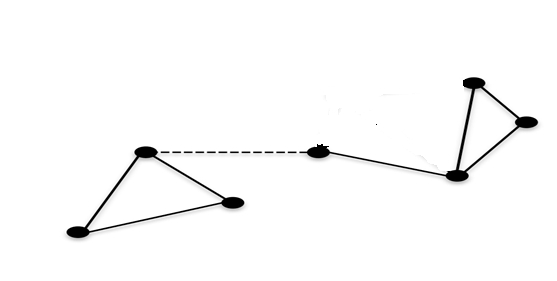# Connectivity, Distance, and Spanning Trees

## Spanning Tree

One simple definition is that a tree is a connected graph associated with no cycles, where a cycle let's us go from a node to itself without repeating an edge.

A spanning tree for a connected graph G is defined as a tree containing all the vertices of G.

Spanning trees are often implemented for Internet routing Algorithms. In the Internet, computers (nodes) are often connected with many redundant physical connections.

Total number of Spanning Trees in a Graph. If a graph is a complete graph with n no. of vertices, then total number of spanning trees is n(n-2)

where n is denoted as the number of nodes in the graph. In complete graph, the task is equal to counting different labelled trees with n nodes for which have Cayley's formula.

## Connectivity

In mathematics and computer science, connectivity is one of the basic concepts of graph theory

it requires the minimum number of elements (nodes or edges) that required to be removed to separate the remaining nodes into isolated subgraphs.

It is closely related to the theory of network flow problems.This graph becomes disconnected when the dashed edge is eliminated.

Vertex Connectivity. The vertex connectivity of a graph is the at least number of nodes whose deletion disconnects it.

Vertex connectivity is sometimes denoted as "point connectivity" or simply "connectivity."

Edge Connectivity. The at least number of edges whose deletion from a graph disconnects, also denoted as the line connectivity.

The edge connectivity of a disconnected graph is 0, while that of a connected graph associated with a graph bridge is 1.

## Distance

The distance between two nodes can be calculated in terms of lowest common ancestor. Following is the formula.

Dist(d1, d2) = Dist(root, d1) + Dist(root, d2) - 2*Dist(root, lca)
'd1' and 'd2' are the two given keys
'root' is root of given Binary Tree.
'lca' is lowest common ancestor of d1 and d2
Dist(d1, d2) is the distance between d1 and d2.

Updated on: 02-Jan-2020

271 Views